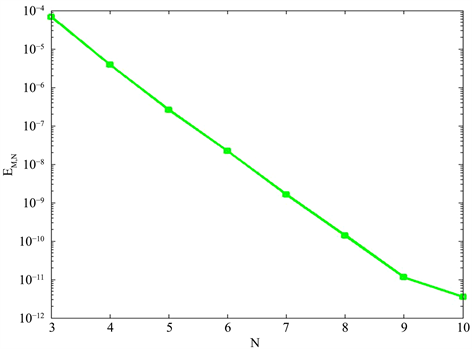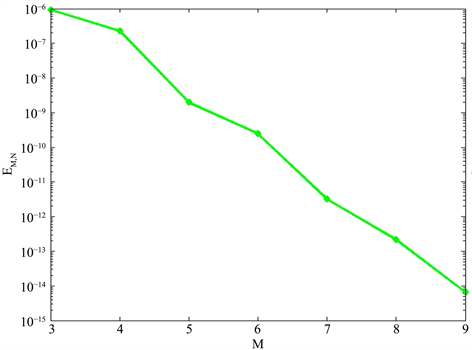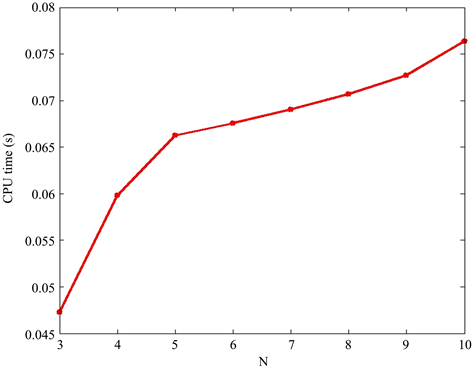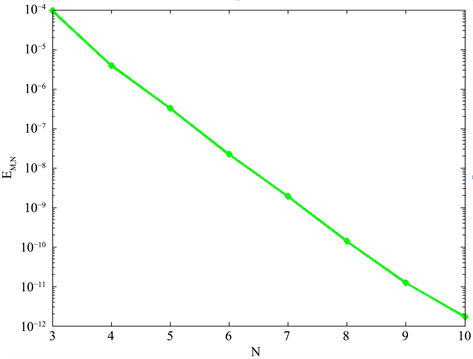# Burgers方程的时空Legendre谱配置方法Space-Time Legendre Spectral Collocation Methods for Burgers Equation

DOI: 10.12677/AAM.2021.104147, PDF, HTML, XML, 下载: 20  浏览: 53  国家自然科学基金支持

Abstract: A Legendre spectral collocation scheme is constructed for Burgers equation by using the Legendre collocation method in time and space, which is a nonlinear system using Lagrange interpolation polynomials. Numerical results demonstrate the efficiency and high accuracy of the proposed algorithm.

1. 引言

Burgers方程由Bateman 1915年在研究流体运动时提出 。浅水波以及一些物理系统中的波动过程可以归结为Burgers方程。一维Burgers方程精确解首先由Benton和Platzman得到 ，因此，求解Burgers方程是科学或工程领域中的重要课题。一些作者用Chebyshev谱–Euler混合方法、高阶紧致有限体积、时空耦合谱元方法和迎风LDQ方法求解Burgers方程初边值问题    。由于时间和空间方向的误差阶不同，导致时间和空间方向的模数不平衡，这就会增加计算工作量，为克服这个局限性，有作者对发展型方程构造了时空谱方法  。最近，一些作者研究了以Legendre-Gauss-Lobatto节点为配置点的微分矩阵的一些性质  ，并用于求解常微分方程定解问题的数值解  ，特别有作者构造了KdV方程Cauchy问题的时空谱配置方法  ，基于这些工作，本文构造Burgers方程初边值问题的时空Legendre谱配置方法，具体地就是考虑如下Burgers方程初边值问题：

$\left\{\begin{array}{ll}{\partial }_{s}u+u{\partial }_{x}u-{\partial }_{x}^{2}u=0,\hfill & x\in \left(-1,1\right),\text{}s\in \left(0,T\right],\hfill \\ u\left(-1,s\right)={g}_{1}\left(s\right),\text{}u\left(1,s\right)={g}_{2}\left(s\right),\hfill & s\in \left[0,T\right],\hfill \\ u\left(x,0\right)=\phi \left(x\right),\hfill & x\in \left[-1,1\right].\hfill \end{array}\text{}$ (1)

2. 基于Gauss节点的插值多项式及其微分矩阵

${L}_{N}\left(x\right)\text{}\left(x\in I=\left(-1,1\right)\right)$ 为N次Legendre多项式。 ${x}_{0}=-1;{x}_{N}=1;\text{}{x}_{k}\left(1\le k\le N-1\right)$${\partial }_{x}{L}_{N}\left(x\right)=0$ 的根 。以 ${x}_{k}$ 为节点的Lagrange插值基函数为：

${\psi }_{k}\left(x\right)=\frac{\left(1-{x}^{2}\right){\partial }_{x}{L}_{N}\left(x\right)}{N\left(N+1\right)\left({x}_{k}-x\right){L}_{N}\left({x}_{k}\right)}.$ (2)

${P}_{N}\left(I\right)$ 为次数不超过 $N$ 的多项式集合，对 $u\left(x\right)\in C\left(\stackrel{¯}{I}\right)$，其Lagrange插值多项式

${p}_{N}\left(x\right)=\underset{j=0}{\overset{N}{\sum }}{u}_{j}{\psi }_{j}\left(x\right),\text{}{u}_{j}=u\left({x}_{j}\right),\text{}x\in I.$

${p}_{N}\left(x\right)$ 关于 $x$$m$ 阶导数，并令 $x={x}_{k},\text{}k=0,1,\cdots ,N$，得

${\partial }_{x}^{m}{p}_{N}\left(x\right)=\underset{j=0}{\overset{N}{\sum }}{u}_{j}{\partial }_{x}^{m}{\psi }_{j}\left(x\right).$

${D}^{\left(m\right)}={\left({\partial }_{x}^{m}{\psi }_{j}\left({x}_{k}\right)\right)}_{0\le k,j\le N},{\begin{array}{cc}& D={D}^{\left(1\right)}=\left({d}_{kj}={\partial }_{x}{\psi }_{j}\left({x}_{k}\right)\right)\end{array}}_{0\le k,j\le N}.$

${d}_{kj}=\left\{\begin{array}{ll}-\frac{N\left(N+1\right)}{4},\hfill & k=j=0;\hfill \\ \frac{{L}_{N}\left({x}_{k}\right)}{\left({x}_{k}-{x}_{j}\right){L}_{N}\left({x}_{j}\right)},\hfill & k\ne j;\hfill \\ \frac{N\left(N+1\right)}{4},\hfill & k=j=N;\hfill \\ 0,\hfill & k=j,\left(k,j=1,2,\cdots ,N-1\right).\hfill \end{array}$ (3)

3. Burgers方程初边值问题的谱配置格式

$\left\{\begin{array}{ll}\frac{2}{T}{\partial }_{t}u+u{\partial }_{x}u-{\partial }_{x}^{2}u=0,\hfill & x\in \left(-1,1\right),\text{}t\in \left(-1,1\right],\hfill \\ u\left(-1,t\right)={g}_{1}\left(s\left(t\right)\right),u\left(1,t\right)={g}_{2}\left(s\left(t\right)\right),\hfill & t\in \left[-1,1\right],\hfill \\ u\left(x,-1\right)=\phi \left(x\right)\hfill & x\in \left[-1,1\right].\hfill \end{array}$ (4)

$\Omega =I\otimes I,$${Q}_{M,N}\left(\Omega \right)={P}_{M}\left(I\right)\otimes {P}_{N}\left(I\right)$。式(4)的Legendre时空谱配置方法就是求 ${u}_{M,N}\left(x,t\right)\in {Q}_{M,N}\left(\Omega \right)$ 满足：

$\left\{\begin{array}{ll}\frac{2}{T}{\partial }_{t}{u}_{M,N}+{u}_{M,N}{\partial }_{x}{u}_{M,N}-{\partial }_{x}^{2}{u}_{M,N}=0,\hfill & x\in \left(-1,1\right),\text{}t\in \left(-1,1\right],\hfill \\ {u}_{M,N}\left(-1,t\right)={g}_{1}\left(s\left(t\right)\right),{u}_{M,N}\left(1,t\right)={g}_{2}\left(s\left(t\right)\right),\hfill & t\in \left[-1,1\right],\hfill \\ {u}_{M,N}\left(x,-1\right)=\phi \left(x\right),\hfill & x\in \left[-1,1\right].\hfill \end{array}$ (5)

${u}_{M,N}\left(x,t\right)=\underset{n=0}{\overset{N}{\sum }}\underset{m=0}{\overset{M}{\sum }}{\stackrel{^}{u}}_{m,n}{\psi }_{n}^{}\left(x\right){\psi }_{m}\left(t\right)$ 逼近(4)式的解，并将其带入到(5)式中，得：

$\left\{\begin{array}{l}\frac{\text{2}}{T}\underset{n=0}{\overset{N}{\sum }}\underset{m=0}{\overset{M}{\sum }}{\stackrel{^}{u}}_{m,n}{\psi }_{n}^{}\left({x}_{k}\right){\partial }_{t}{\psi }_{m}\left({t}_{l}\right)+\underset{n=0}{\overset{N}{\sum }}\underset{m=0}{\overset{M}{\sum }}{\stackrel{^}{u}}_{m,n}{\psi }_{n}^{}\left({x}_{k}\right){\psi }_{m}\left({t}_{l}\right)\underset{n=0}{\overset{N}{\sum }}\underset{m=0}{\overset{M}{\sum }}{\stackrel{^}{u}}_{m,n}{\partial }_{x}{\psi }_{n}^{}\left({x}_{k}\right){\psi }_{m}\left({t}_{l}\right)\\ -\underset{n=0}{\overset{N}{\sum }}\underset{m=0}{\overset{M}{\sum }}{\stackrel{^}{u}}_{m,n}{\partial }_{x}^{2}{\psi }_{n}^{}\left({x}_{k}\right){\psi }_{m}\left({t}_{l}\right)=0,\text{}k=1,2,\cdots ,N-1;l=1,2,\cdots ,M,\\ \underset{n=0}{\overset{N}{\sum }}\underset{m=0}{\overset{M}{\sum }}{\stackrel{^}{u}}_{m,n}{\psi }_{n}^{}\left({x}_{0}\right){\psi }_{m}\left({t}_{l}\right)={g}_{1}\left(\frac{{t}_{l}+1}{2}T\right),\underset{n=0}{\overset{N}{\sum }}\underset{m=0}{\overset{M}{\sum }}{\stackrel{^}{u}}_{m,n}{\psi }_{n}^{}\left({x}_{N}\right){\psi }_{m}\left({t}_{l}\right)={g}_{2}\left(\frac{{t}_{l}+1}{2}T\right),\\ l=0,1,\cdots M,\text{}\underset{n=0}{\overset{N}{\sum }}\underset{m=0}{\overset{M}{\sum }}{\stackrel{^}{u}}_{m,n}{\psi }_{n}^{}\left({x}_{k}\right){\psi }_{m}\left({t}_{0}\right)=\phi \left({x}_{k}\right),\text{}k=0,1,2,\cdots ,N.\end{array}$ (6)

${\stackrel{^}{d}}_{l,m}^{}={\partial }_{t}^{}{\psi }_{m}\left({t}_{l}\right),$ ${\stackrel{˜}{d}}_{k,n}={\partial }_{x}^{}{\psi }_{n}\left({x}_{k}\right),\text{}{\stackrel{˜}{d}}_{k,n}^{\left(\text{2}\right)}={\partial }_{x}^{\text{2}}{\psi }_{j}\left({x}_{k}\right)$，利用Lagrange插值多项式的性质，式(6)关于 $l$$k$ 展开，则(6)式可转化成矩阵形式为：

$AX-X{B}^{\text{T}}+X.*D=-X.*\left(X{C}^{\text{T}}\right)-F.$ (7)

${E}_{n}$ 表示 $n$ 阶单位矩阵，“ $\otimes$ ”表示Kronecker积， $G$ 是矩阵 $D$ 按行向量拉长后的转置向量， $R$ 是矩阵 $F$ 按行向量拉长后的转置向量，“ $.*$ ”表示对应元素相乘，则(7)式可化为如下的非线性方程组：

$\left(A\otimes {E}_{N-1}-{E}_{M}\otimes B\right)Y+Y.*G=-Y\text{.*}\left(\left({E}_{M}\otimes C\right)Y\right)-R.$ (8)$\begin{array}{l}Y={\left({\stackrel{^}{u}}_{1,1},{\stackrel{^}{u}}_{1,2},\cdots ,{\stackrel{^}{u}}_{1,N-1},{\stackrel{^}{u}}_{2,1},{\stackrel{^}{u}}_{2,2},\cdots ,{\stackrel{^}{u}}_{2,N-1},\cdots ,{\stackrel{^}{u}}_{M,1},{\stackrel{^}{u}}_{M,2},\cdots ,{\stackrel{^}{u}}_{M,N-1}\right)}^{\text{T}},\\ {Y}_{0}={\left({\stackrel{^}{u}}_{0,1},{\stackrel{^}{u}}_{0,2},\cdots ,{\stackrel{^}{u}}_{0,N-1},0,0,\cdots ,0,\cdots ,0,0,\cdots ,0\right)}^{\text{T}},\\ R={\left({f}_{1,1},{f}_{1,2},\cdots ,{f}_{1,N-1},{f}_{2,1},{f}_{2,2},\cdots ,{f}_{2,N-1},\cdots ,{f}_{M,1},{f}_{M,2},\cdots ,{f}_{M,N-1}\right)}^{\text{T}},\\ G={\left({d}_{1,1},{d}_{1,2},\cdots ,{d}_{1,N-1},{d}_{2,1},{d}_{2,2},\cdots ,{d}_{2,N-1},\cdots ,{d}_{M,1},{d}_{M,2},\cdots ,{d}_{M,N-1}\right)}^{\text{T}}.\end{array}$

4. 数值结果

Burgers方程有精确孤波解及初边值 ：

$\begin{array}{l}u\left(x,s\right)=\frac{2\frac{\omega }{k}{\text{e}}^{\frac{-\omega }{\alpha {k}^{2}}\left(kx-\omega s\right)}}{{C}_{1}+{\text{e}}^{\frac{-\omega }{\alpha {k}^{2}}\left(kx-\omega s\right)}},\text{}\phi \left(x\right)=\frac{2\frac{\omega }{k}{\text{e}}^{\frac{-\omega }{\alpha {k}^{2}}kx}}{{C}_{1}+{\text{e}}^{\frac{-\omega }{\alpha {k}^{2}}kx}},\\ {g}_{1}\left(s\right)=\frac{2\frac{\omega }{k}{\text{e}}^{\frac{-\omega }{\alpha {k}^{2}}\left(-k-\omega s\right)}}{{C}_{1}+{\text{e}}^{\frac{-\omega }{\alpha {k}^{2}}\left(-k-\omega s\right)}},\text{}{g}_{2}\left(s\right)=\frac{2\frac{\omega }{k}{\text{e}}^{\frac{-\omega }{\alpha {k}^{2}}\left(k-\omega s\right)}}{{C}_{1}+{\text{e}}^{\frac{-\omega }{\alpha {k}^{2}}\left(k-\omega s\right)}}.\end{array}$ (9)

${E}_{M,N}=\underset{1\le l\le M,1\le k\le N-1}{\mathrm{max}}|{u}_{M,N}\left({x}_{k},{t}_{l}\right)-u\left({x}_{k},{t}_{l}\right)|.$Figure 1. ${L}^{\infty }-$ error with $M=\text{7},k=\text{0}\text{.5},\omega =0.3,{C}_{1}=2\omega /k,\alpha =1$Figure 2. ${L}^{\infty }-$ error with $N=15,k=\text{0}\text{.5},\omega =\text{0}\text{.3},{C}_{1}=2\omega /k,\alpha =1$Figure 3. Costing seconds of CPU with $M=\text{7},k=\text{0}\text{.5},\omega =\text{0}\text{.3},{C}_{1}=2\omega /k,\alpha =1$Figure 4. ${L}^{\infty }-$ error with $M=\text{20},k=\text{0}\text{.5},\omega =\text{0}\text{.3},{C}_{1}=2\omega /k,\alpha =1$

5. 结论

  Burger, J.M. (1948) A Mathematical Model Illustrating the Theory of Turbulence. In: Von Mises, R. and Von Karman, T., Eds., Advances in Applied Mechanics, Academic Press, New York, 171-199. https://doi.org/10.1016/S0065-2156(08)70100-5  Benton, E. and Platzman, G.W. (1972) A Table of Solutions of the One-Dimensional Burgers’ Equation. Quarterly of Applied Mathematics, 30, 195-212. https://doi.org/10.1090/qam/306736  闵涛, 任菊成, 耿蓓, 等. Chebyshev谱-Euler混合方法求解一类非线性Burgers方程[J]. 数值计算与计算机应用, 2013, 34(2): 3-10.  高巍, 张宝, 李宏, 刘洋. Burgers方程的高阶紧致有限体积解法[J]. 应用数学, 2016, 29(2): 331-339.  王亚洲, 秦国良, 和文强, 包振忠. 时空耦合谱元方法求解一维Burgers方程[J]. 西安交通大学学报, 2017, 51(1): 45-50.  陈龙, 张学莹, 胡婷婷. 基于迎风LDQ方法解Burgers方程[J]. 西北师范大学学报, 2017, 53(6): 10-15.  Tang, J.G. and Ma, H.P. (2007) A Legendre Spectral Method in Time for First-Order Hyperbolic Equations. Applied Numerical Mathematics, 57, 1-11. https://doi.org/10.1016/j.apnum.2005.11.009  Liu, L. and Ma, H.P. (2020) Space-Time Spectral Method for Parabolic Inverse Problem with Unknown Control Parameter. Journal of Numerical Methods and Computer Applications, 41, 19-26.  Shen, J., Tang, T. and Wang, L.L. (2011) Spectral Methods: Algorithms, Analysis and Applications. Springer-Verlag, Berlin.  王天军, 殷政伟. Legendre-Gauss-Lobatto节点的一个注记[J]. 河南科技大学学报: 自然科学版, 2012, 33(1): 71-74.  Wang, Z.Q. and Guo, B.Y. (2012) Legendre-Gauss-Radau Collocation Method for Solving Initial Value Problems of First Order Ordinary Differential Equations. Journal of Scientific Computing, 52, 226-255. https://doi.org/10.1007/s10915-011-9538-7  王天军. 一类线性奇异边值问题的谱配置方法[J]. 河南科技大学学报: 自然科学版, 2013, 34(6): 75-78.  Jia, H.L. and Wang, Z.Q. (2013) Chebyshev-Hermite Spectral Collocation Method for KdV Equations. Communications on Applied Mathematics and Computation, 27, 1-8.  马亚楠, 王天军, 李冰冰. Korteweg-de Vries方程的时空谱置方法[J]. 数值计算与计算机应用, 2020. (接收)  杨先林. Burgers方程的精确解[J]. 动力学与控制学报, 2006, 4(4): 308-311.